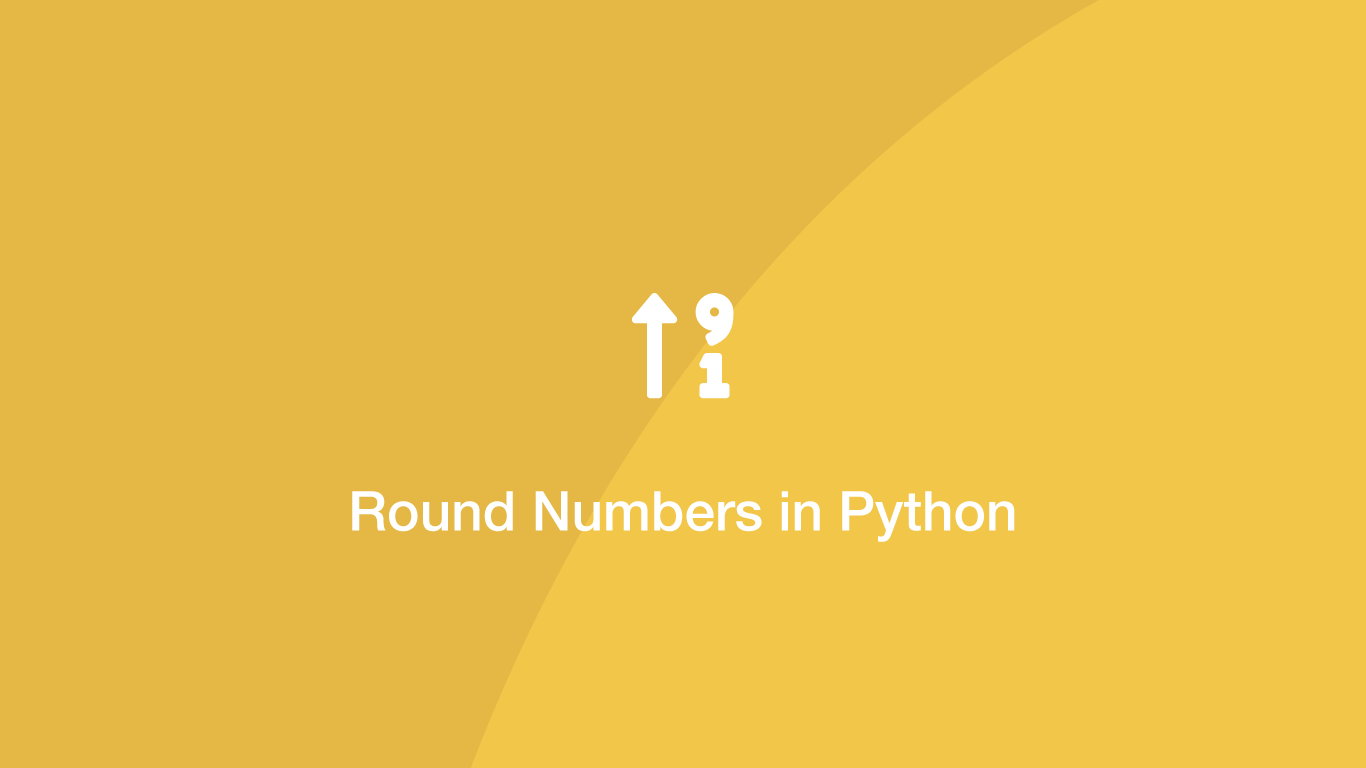# How to Round Numbers Up and Down in PythonIt is common to have numbers that need to be rounded in Python. Fortunately, there is a native Python `round()` function for rounding decimal/floating-point numbers to their nearest integer and a `math` package with methods for always rounding up or down to a whole number.

In this tutorial, we will learn how to round numbers in Python in a variety of different ways with examples.

## Round to the Nearest Integer

To round to the nearest whole number, supply the decimal/floating-point number inside the `()` (parenthesis) of the `round()` function. In the example below, we will store the output from `round()` in a variable before printing it.

``````# round to nearest integer

rounded = round(1.35)

print(rounded)
``````
``````1
``````

The nearest integer to `1.35` is `1` and when we put that float into `round()`, `1` was indeed returned.

## Round Float Up

To always round a number up to an integer regardless of whether it is the closest whole number we can use the `ceil()` method, which is available on the math package. To use it, import the math package and then pass the number to round up in the `()` (parenthesis) or `math.ceil()`.

``````# round float up

import math

result = math.ceil(1.35)

print(result)
``````
``````2
``````

`2` is not the closest integer to `1.35` but `ceil()` rounded it up anyway.

## Round Float Down

To always round a number down, use the `floor()` method of the math package passing the number to round inside the `()` (parenthesis) of `math.floor()`.

``````# round float down

import math

result = math.floor(1.75)

print(result)
``````
``````1
``````

In the example above, `math.floor()` rounded `1.75` down to `1`.

## Round to a Fixed Number of Decimal Places

To round to a decimal place on a number, pass the level to round to as the second argument of the `round()` function.

``````# round to a fix decimal place

rounded = round(1.35, 1)

print(rounded)
``````
``````1.4
``````

In the example above we are essentially rounding `35` as that is the first decimal place in `1.35`.

## Round all Values in a Python list

To round all the values in a list we can loop through the list of numbers and round each value before appending it to a new array.

``````# round all numbers in a list

numbers = [1.23, 4.435, 2.532, 6.2, 1.6]

rounded_list = []

for num in numbers:
rounded_list.append(round(num))

print(rounded_list)
``````
``````[1, 4, 3, 6, 2]
``````

## Using list Comprehension

A shorter way to round a list and create a new one containing those values is to use list comprehension. Let's perform the exact same task ask above with list comprehension.

``````# round list numbers with list comprehension

numbers = [1.23, 4.435, 2.532, 6.2, 1.6]

rounded_list = [round(num) for num in numbers]

print(rounded_list)
``````
``````[1, 4, 3, 6, 2]
``````

## Conclusion

You know now how to round numbers in Python in a variety of different ways depending on how the rounding should behave.

#### Related Tutorials### How to Round Decimals Up and Down in JavaScript

September 08, 2020### How to Always Round-Up a Number in Python

November 22, 2021### Truncate Float in Python

June 29, 2021### How to Convert a String to a Number in JavaScript

August 20, 2020### How to Divide in Python

April 01, 2021### How to get Random Elements from an Array in JavaScript

September 13, 2020